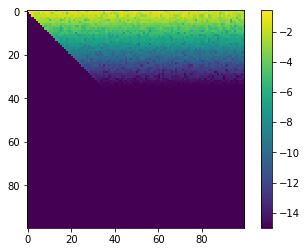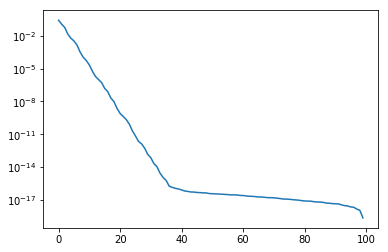# Rank-Revealing QR¶

Note: scipy.linalg, not numpy.linalg!

In :
import numpy as np
import scipy.linalg as la
import matplotlib.pyplot as pt


## Obtain a low-rank matrix¶

In :
n = 100
A0 = np.random.randn(n, n)
U0, sigma0, VT0 = la.svd(A0)
print(la.norm((U0*sigma0)@VT0 - A0))

sigma = np.exp(-np.arange(n))

A = (U0 * sigma).dot(VT0)

2.654266110213982e-13


## Run the factorization¶

Compute the QR factorization with pivoting=True.

In :
Q, R, perm = la.qr(A, pivoting=True)


First of all, check that we've obtained a valid factorization

In :
la.norm(A[:, perm] - Q@R, 2)

Out:
6.317156500391685e-16
In :
la.norm(Q@Q.T - np.eye(n))

Out:
7.773451587511033e-15

Next, examine $R$:

In :
pt.imshow(np.log10(1e-15+np.abs(R)))
pt.colorbar()

Out:
<matplotlib.colorbar.Colorbar at 0x7f33a92b6fd0>Specifically, recall that the diagonal of $R$ in QR contains column norms:

In :
pt.semilogy(np.abs(np.diag(R)))

Out:
[<matplotlib.lines.Line2D at 0x7f33ad9f27d0>]• In the case of scipy's transform, diagonal entries of $R$ are guaranteed non-increasing.
• But there is a whole science to how to choose the permutations (or other source vectors)
• and what promises one is able to make as a result of that
In [ ]: